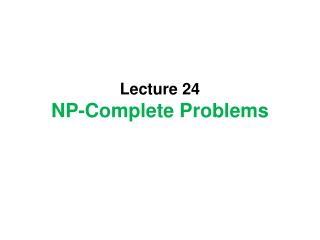# Lecture 24 NP-Complete Problems - PowerPoint PPT PresentationDownload PresentationLecture 24 NP-Complete Problems

Lecture 24 NP-Complete ProblemsDownload Presentation## Lecture 24 NP-Complete Problems

- - - - - - - - - - - - - - - - - - - - - - - - - - - E N D - - - - - - - - - - - - - - - - - - - - - - - - - - -
##### Presentation Transcript

1. Lecture 24NP-Complete Problems

2. (1) Polynomial-time many-one reduction

3. A < m B p • A set A in Σ* is said to be polynomial-time many-one reducible to B in Γ* if there exists a polynomial-time computable function f: Σ* → Γ* such that x ε A iff f(x) ε B.

4. A = Hamiltonian cycle (HC) • Given a graph G, does G contain a Hamiltonian cycle?

5. B = decision version of Traveling Salesman Problem (TSP) • Given n cities and a distance table between these n cities, find a tour (starting from a city and come back to start point passing through each city exactly once) with minimum total length. • Given n cities, a distance table and k > 0, does there exist a tour with total length < k?

6. HC <m TSP p • From a given graph G, we need to construct (n cities, a distance table, k).

7. SAT < m 3-SAT p • SAT: Given a Boolean formula F, does F have a satisfied assignment? • An assignment is satisfied if it makes F =1. • 3-SAT: Given a 3-CNF F, does F have a satisfied assignment?

8. Property of < m p p p p • A <m B and B <m C imply A <m C • A <m B and B ε P imply A ε P p

9. NP-complete • A set A is NP-hard if for any B in NP, B <m A. • A set A is NP-complete if it is in NP and NP-hard. • A decision problem is NP-complete if its corresponding language is NP-complete. • An optimization problem is NP-hard if its decision version is NP-hard. p

10. (2) Cook Theorem SAT is NP-complete

11. Proof of Cook Theorem

12. The 1st tape should be

13. The last tape should contain The final state.

14. Exercise!!!

15. 3-SAT is NP-complete